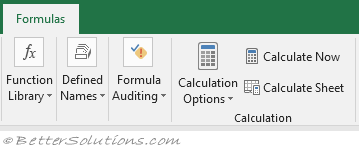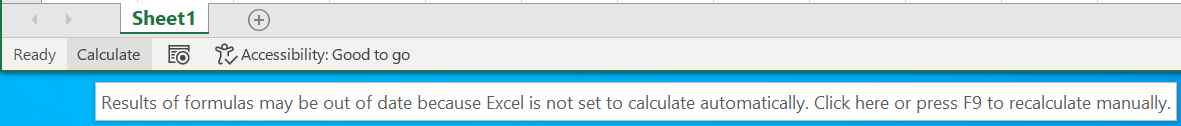# CalculationA workbook can be updated (or calculated) when it is opened, closed.
You can also normally interrupt the calculation process by pressing Escape several times.
(F9) - Smart Calculation. Calculates All the worksheets in All the open workbooks (only cells containing formulas that have changed since the last calculation, will be updated). [Application.Calculate]
(Shift + F9) - Smart Calculation. Calculates the Active / Selected worksheets in the Active workbook (only cells containing formulas that have changed since the last calculation, will be updated). [Sheets(1).Calculate]
(Ctrl + Alt + F9) - Full Calculation. Calculates All the worksheets in All the open workbooks. (all cells containing formulas). [Application.CalculateFull]
(Ctrl + Alt + Shift + F9) - Full Recalculation. Calculates All the worksheets in All the open workbooks (all cells containing formulas and rebuilding the dependency tree and calculation chain). [Application.CalculateFullRebuild]

There is currently no way to quickly perform a full calculation of all the cells in just the active workbook.
(Ctrl + Shift + F9) - This is not a default shortcut key but some third party add-ins use this for performing a full calculation of all the cells in just the active workbook.

### Options > Formulas Tab

The File > Options - Formulas Tab contains all the options for controlling formula calculation.

### Automatic Calculation

The default setting is Automatic this means that Excel will automatically recalculate all the necessary cells in a workbook when a value is changed.
This type of calculation is the default and is what some people call a minimum recalculation.
This means that the data and formulas in your workbook are constantly up-to-date.
Most of the time when the workbook is fairly simple recalculation takes a fraction of a second since the majority of the cells will be unaffected.
When you have a complicated workbook containing a lot of formulas, Excel will determine which cells to recalculate and in what order.
It is important to remember though that Excel can only track 65,536 dependencies to unique references.
If Excel finds more than 65,536 dependencies then the whole workbook is calculated and the word "Calculate" may remain in the status bar - check

### Manual calculation

This lets you select or clear the Recalculate Before Save check box.
The "Recalculate Workbook Before Save" check box controls if the formulas are recalculated before the workbook is closed.
This is often a good idea, as it prevents a user opening the workbook to display data that is not up-to-date.
Manual calculation is a good idea when you have large workbooks as you want to control when a recalculation occurs.
If your workbook contains any uncalculated formulas in the active workbook when you are working in Manual calculation the word "Calculate" will be displayed in the status bar.### Why does my Calculation change to Manual ?

Your calculation setting (and your iteration setting) is actually an Application Setting rather than a workbook setting.
However despite this being an application setting it is saved into your individual workbooks.
The calculation settings are taken from the first workbook you open (in that session) and then ignored in subsequent workbooks.
Depending on the order in which you open your workbooks your settings may change.
For example lets say that all of your workbooks have been saved with a calculation settings of Automatic.
This works fine for you until by chance the first workbook you open in your session happens to be a workbook created by someone else (who by mistake has saved it with manual calculation).
In this scenario your calculation settings will be set to manual and when you open up your own workbooks the calculation will still be set to manual.

### Important

For information about Iteration and Circular References, please refer to the Circular References page.
Calculation is not a workbook or worksheet specific setting. When you change this setting it will affect all open workbooks (and worksheets).